TL;DR: Create a look up table which defines the order. Then, create a calculated column in the main table which retrieves the order from this look up, and sort the target column using the calculated column.

## Problem

So, say we had a table which contains people's birth month and year:If we sort by name: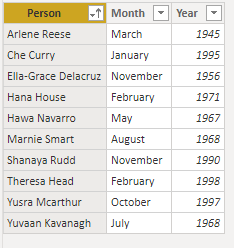Or by year: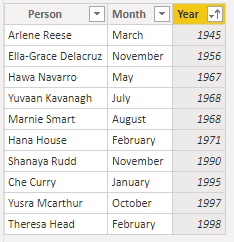Then we get the expected results. However, if we sort by month: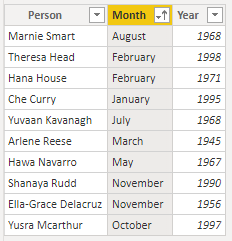We clearly would expect different behaviour. To alter the sort order, we need to define an order column in the table and tell the month column to instead sort based on that order. We need to create the following column: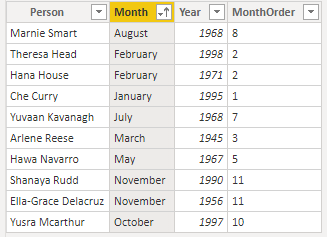And then sort the month column by its order: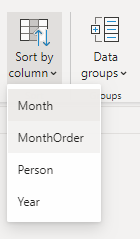So, we could try doing this with a calculated column: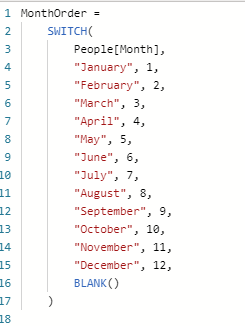However, when we try to sort on the created column, we get this error:If we instead create a new column using the query editor, we can avoid this circular reference. However, the problem with this approach is that Power Query doesn't have a built-in `SWITCH` function, so you end up with a large number of `IF` statements. This can get quite unwieldy if you have many different values to compute.

## Solution

So, instead, we can define the column using relationships. To achieve this, we create a look-up table: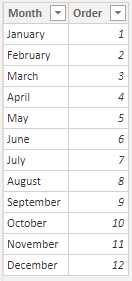This table needs to be created in the Power Query editor, not calculated from the original table, otherwise you end up with another circular reference. We then create a relationship between the look-up and original tables (sometimes this will have been detected for you):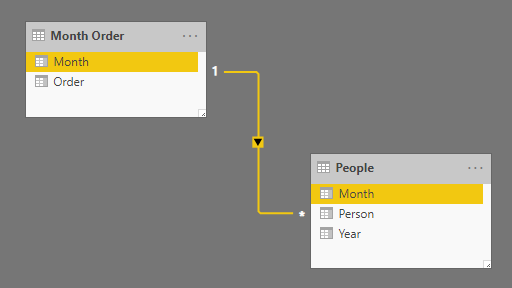And then create a calculated column using the lookup: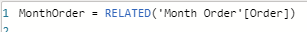We can then sort the `Month` column by our calculated column, and the behaviour will work as expected: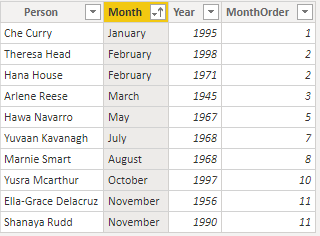### Carmel Eve

#### Software Engineer I